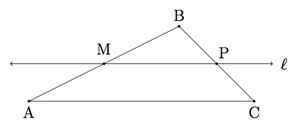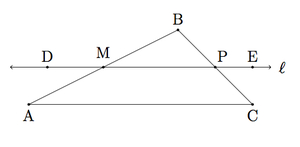# Joining two midpoints of sides of a triangle

Alignments to Content Standards: G-SRT.B.4

Suppose $ABC$ is a triangle. Let $M$ be the midpoint of $\overline{AB}$ and $\ell$ the line through $M$ parallel to $\overleftrightarrow{AC}$:1. Show that angle $CAB$ is congruent to angle $PMB$ and that angle $BPM$ is congruent to angle $BCA$. Conclude that triangle $MBP$ is similar to triangle $ABC$.
2. Use part (a) to show that $P$ is the midpoint of $\overline{BC}$.

## IM Commentary

This task is closely related to very important material about similarity and ratios in geometry. In this case, the point $M$ selected on the triangle $ABC$ is the midpoint of $\overline{AB}$. More generally, consider the picture below:Here $F$ is any point on $\overline{AB}$ (other than one of the endpoints) and $G$ is the point on $\overline{BC}$ so that $\overleftrightarrow{FG}$ parallel to line $\overleftrightarrow{AC}$. Then we have the relationship $$\frac{|FA|}{|AB|} = \frac{|GC|}{|BC|}.$$ The converse of this result holds as well: if $F$ is a point on $\overline{AB}$ and $G$ is a point on $\overline{BC}$ so that the above equality holds then then $\overleftrightarrow{FG}$ is parallel to $\overleftrightarrow{AC}$.

The argument presented here for midpoints works also in the more general setting mentioned in the above paragraph. Essential to this argument is the use of the AAA criterion for triangle similarity, G-SRT.3. The task can be used for assessment provided students are aware that they may use the AAA result in part (b) of this problem.

## Solution

1. We require two auxiliary points on line $\ell$: a point $D$ so that $M$ is between $P$ and $D$ and a point $E$ so that $P$ is between $M$ and $E$ as in the picture belowLines $\ell$ and $\overleftrightarrow{AC}$ are parallel and $\overleftrightarrow{AB}$ is a transverse for these parallel lines. Therefore angle $CAB$ is congruent to angle $DMA$ since these are alternate interior angles for a transverse meeting parallel lines. Angle $DMA$ is congruent to angle $PMB$ by the vertical angle theorem. So angle $CAB$ is congruent to angle $PMB$.

The same argument will show that angles $BPM$ and $BCA$ are congruent: angle $BCA$ is congruent to angle $EPC$ (alternate interior angles) and angle $EPC$ is congruent to angle $BPM$ (vertical angles) establishing that angles $BCA$ and $BPM$ are congruent.

We have $m(\angle ABC) = m(\angle MBP)$ since these are the same angles. Therefore the triangles $MBP$ and $ABC$ share three congruent angles and they are similar triangles. Alternatively, we could stop after producing two congruent angles and use the AA criterion for similarity of triangles.

2. Since triangles $MBP$ and $ABC$ are similar it follows that $$\frac{|PB|}{|CB|} = \frac{|BM|}{|BA|}.$$ Since $M$ is the midpoint of $\overline{BA}$, we have $\frac{|BM|}{|BA|} = \frac{1}{2}$ and so $\frac{|PB|}{|CB|} = \frac{1}{2}$ which means that $P$ is the midpoint of segment $\overline{CB}$.